Hypersphere CosmologyAlternative to big-bang

Wednesday, 02 July 2014 16:50

HyperSphere Cosmology

Hypersphere Cosmology in a Nutshell

1a) The Universe consists of a Hypersphere (a 3-Sphere or 4-ball) where: -alsoalsoThe Universe thus has an ‘orbital’ velocity of lightspeed and an escape velocity ofHyperspheres can never have a radiusof less thanHyperspheres may form inside black holes but singularities do not exist.

1b) The magnitudes of G and c arise from the structure of the entire universe as in Mach’s principle: -

G =also=1c) The Anderson deceleration arises as a consequence of the gravity of the entire universe: -= A     also  A =The Anderson deceleration actually represents the small positive curvature of the Universe.

Key.

M = Mass of the Universe. L = Antipode length. A = Anderson deceleration.= radius of hypersphere.

Anderson deceleration = Pioneer deceleration –Thermal Recoil. This model predicts that it will bottom out at around 6.9 x 10^-10 m/s^-2.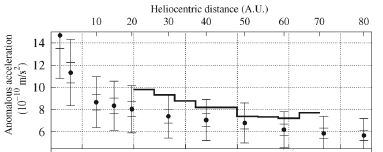2a) The Universe undergoes a fourth dimensional rotation or ‘vorticitation’ which gives it an angular velocity W, shown by: -=This equation derives from Gödel, but for a hypersphere rather than a sphere, wherethe volume of the 3d ‘surface’ of the hypersphere equals= 2also2b) The Universe thus has a ‘centrifugal’ acceleration ofwhich exactly balances its ‘centripetal’ acceleration, the Anderson deceleration, thus imposing an omnidirectional resistance to linear motion.

2c) The universe has a frequencyof vorticitation where: -=This equates to the time taken for complete 4d ‘rotation’, during which the Universe rotates into an antimatter phase and back again. This implies an angular rotation of only 0.0056 Arcseconds per century only. We have not noticed this yet.

3) Redshift (Z) arises as the small positive curvature of the Universe resists the passage of light.= Observed wavelength.=  Expected wavelength.

(d = astronomical distance, A = Anderson acceleration)The Universe does not expand.

4) The positive spacetime curvature the Universe increases orbital velocity: -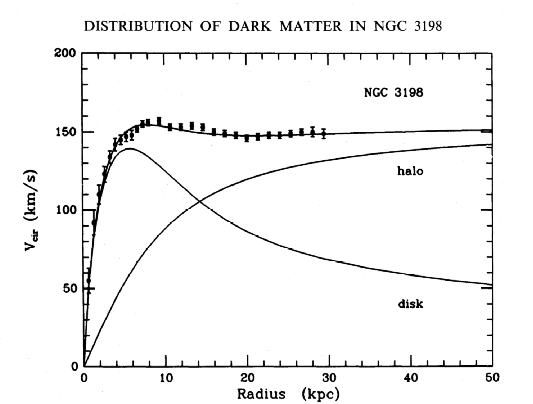By the equation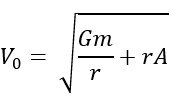As the general form of disc galaxy rotation curves beyond the central bulge takes the form of v = sqrt(1/r + r) as below:-

Blue, v = sqrt(1/r). Red, v = sqrt(r). Green, v = sqrt(1/r + r)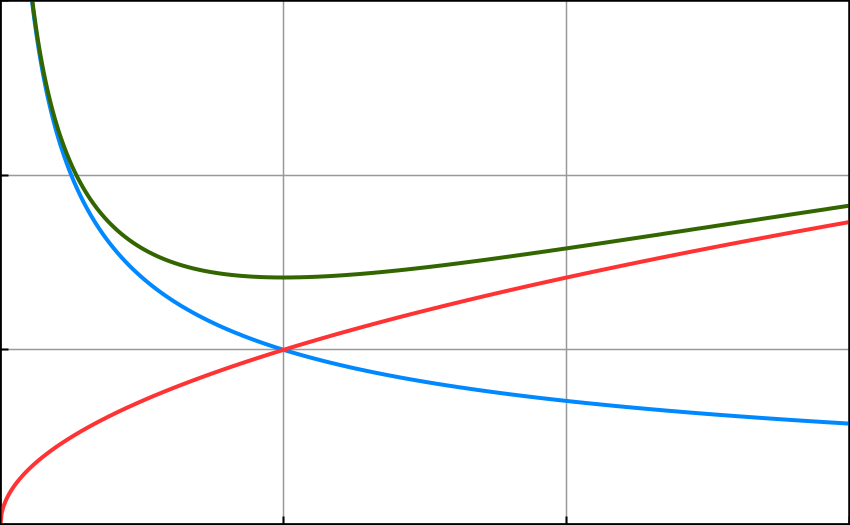The positive spacetime curvature increases centripetal acceleration which increases orbital velocity flattening galactic rotation curves, this repays the energy that the curvature takes from linear motions.

Dark matter does not exist.

5) The positive curvature of the Universe has a lensing effect on the passage of light across it: -=Where= hyperspherical lensing and d stands for the ratio a/L, astronomical distance over antipode distance. . The lensing distorts apparent magnitude and thus creates a mismatch with redshift leading to the dubious assumption of an expanding acceleration.The vertical axis for a number of factors runs from zero to three, marked in divisions of 0.5 with the unity line highlighted in purple for clarity. The horizontal axis runs from observer to antipode.

The red line shows redshift Z, where Z = (c/(c-(dA)^0.5)-1 where d = astronomical distance.

Note that a redshift of 1 at about 7 billion light years denotes the halfway point to the antipode distance. Redshift climbs exponentially towards infinity at the antipode; observations become increasingly difficult up to redshift 10 and then virtually impossible beyond.

The yellow line represents schematically the hyperspherical geodesic from the observer to the observer’s antipode; the curved path that light actually takes in the cosmic hypersphere.

The blue line represents schematically the observer’s assumed sight line for flat space.

The green line represents schematically the difference between the actual and the assumed sight line, and thus the degree of Hyperspherical Lensing LH, that light becomes subject to at various distances. Note that in this revised version of the model, the line has the inverse configuration to previous models on this site and the equation governing it has the form

LH  = 1/(1+((d-d^2)^0.5))-d) where here, d = astronomical distance/antipode distance.

The negative lensing at distances below 7 billion light years explains the anomalously low luminosity of type 1A supernovae without recourse to the hypothesis of an accelerating expansion driven by some mysterious dark energy.

The positive lensing at distances greater than 7 billion light years explains the increase in angular size of very distant structures without recourse to the hypothesis of an expanding universe at all.

Dark energy does not exist.

Thus the Universe consists of a hypersphere, finite but unbounded in both space and time and constant in size, and vorticitating with a small positive spacetime curvature, despite the apparent temporal and spatial horizons.

Inflation did not occur.

6) The Cosmic Microwave Background Radiation. The CMBR consists of trans-antipodal starlight which has gone right round the universe, perhaps many times, until it has become redshifted till it achieves thermodynamic equilibrium with the thin intergalactic medium.

7) Antimatter.  The apparent absence of substantial quantities of antimatter in this universe arises because time rather than space separates matter from antimatter. Due to the 4d vorticitation of the Universe, all matter in the Universe will have an opposite sign to all matter about after a time corresponding to the temporal
horizon of the universe

8) Entropy. The entropy/information of the universe remains constant and proportional to its surface area in accordance with the Beckenstein-Hawking conjecture. The dimensions of the Universe all come out at the same multiple U, the ubiquity constant, of the Planck quantities: -

U =====~The Universe thus has an information deficit leading to an effective quantisation of space and time at about: -and atIf we take T, the temporal horizon, as equivalent to the time elapsed since the big bang in conventional cosmology then the predicted quantisation of spacetime comes out at:-

Planck length and Planck time x 5.4 x 10^20.

Holometry experiments in progress may show this.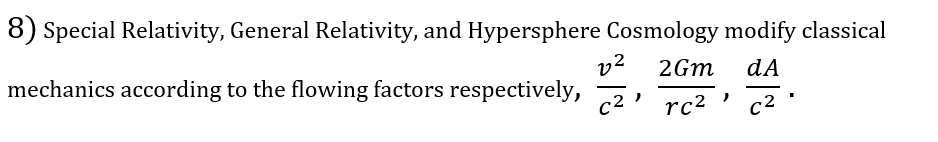9) Metallicity and Black Hole build-up.

Cosmologists refer to all the chemical elements heavier than hydrogen (and sometimes helium as well) as ‘metals’ as the majority of them have metallic qualities. These elements get formed in stars by nuclear fusion (although according to BB theory a lot of the helium got made at the BB.) Now if stars continually fuse hydrogen to heavier elements and no mechanism to break those heavier elements back down exists, then the Perfect Cosmological Principle implied by a finite and unbounded universe hyperspherical in space and time that should appear pretty much the same on the large scale at all points in space and time, seems violated.

The Perfect Cosmological Principle also becomes violated if all the matter in the universe will eventually become sucked into black holes from which it can never escape.

Several observed and hypothesised phenomena may solve the metallicity and black hole problems.

Neutron stars almost certainly exist. Metallic elements falling into neutron stars will get broken back down into neutrons. Neutrons which escape into space rapidly undergo beta decay creating electrons and protons which recombine eventually to form hydrogen. But can they escape?

Pair-Instability Supernovae may well occur. In these events the gravitational collapse of a sufficiently heavy star results in a very powerful gamma ray flux in the core. The gamma rays interact with matter particles initiating electron-positron pair production; this causes the pressure to drop in the core and further collapse to occur. This initiates electron-positron (matter-antimatter) annihilation which explodes the star completely into space leaving no remnant core. Astronomers have observed several possible examples of such an event.

Neutrons may act as Marjorama rather than as Dirac fermions under extreme conditions. In other words they may act as their own antiparticles and annihilate to gamma rays under conditions of extreme compression and temperature.

We do not know what mechanism creates Gamma Ray Bursts, but about once a day one goes off somewhere in the universe. These titanic GRB explosions appear to liberate the energy equivalent of the masses of entire planets or entire stars in brief moments.

Massive and very dense objects of multiple solar masses seem to exist in the universe and most galaxies appear to contain objects of thousands or millions of solar masses at very high densities in their cores. Whether any of these objects have actually formed black holes remains undecided. The maths breaks down at black holes and we cannot directly observe them.

Black holes may not actually exist at all if some mechanism like the above or perhaps some other mechanism limits their maximum density and consigns them to eventually explode, endlessly recycling the matter of the universe.

(Note also that the abandonment of the BB nucleosynthesis model would solve the cosmic lithium abundance problem.)

10) Evidence for the non-expansion of the Universe. The average frequency of quasars at around 6bn light years matches that of quasars at around 10bn light years. If the universe had actually expanded then one would expect some time dilation. See http://phys.org/news190027752.html

- 'There is however surprisingly little direct evidence that the universe is expanding'

11) Conclusions.

The Hypersphere Cosmology model has the virtue of simplicity. It does not require an initial spacetime singularity, or cosmic inflation, or dark matter or dark energy as interpretations of existing observations; it just has a curved geometry and a topology that creates the optical illusion of such things.

It implies that mechanisms  exist for reversing the conventionally expected increase of metallicity through nuclear fusion over time and the build-up of black holes in the universe.

It does not explain how the universe attained its present condition, but the initial conditions of the big bang theory remain similarly inexplicable. Hypersphere Cosmology may not even require initial conditions. We have no good reason to consider non-existence as somehow more fundamental than existence.

Created by Peter J Carroll

This email address is being protected from spambots. You need JavaScript enabled to view it.

20/11/15

see the full viXra paper here http://vixra.org/pdf/1601.0026v1.pdf

summary version here http://vixra.org/abs/1812.0155

Read 338266 times Last modified on Monday, 25 March 2019 18:05
More in this category: Hypersphere Redshift »
• HyperSphere Cosmology +Hypersphere Cosmology in a Nutshell   1a) The Universe consists of a Hypersphere (a 3-Sphere or 4-ball) where: -       also
• Hypersphere Redshift +The alternative mechanism for redshift works as follows: Redshift = Z =   - 1 Where = observed wavelength, = expected wavelength, and the -1
• Hypersphere Rotation +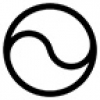The Gödel 3d rotating universe has this solution derived from General Relativity: - “Matter everywhere rotates relative to the compass of
• Hypersphere Holometry - A Prediction +As a consequence of the hypothesis that the universe exists as a hypersphere where   the main parameters of the
• VHC. Vorticitating Hypersphere Cosmology +The overwhelming majority of university and state funded cosmologists believe that the observable universe has expanded from a much smaller
• Hypersphere Visualisation & Lensing +This paper provides a method of visualising the four dimensional hypersphere as a perspective construction in three dimensions, and it
• Sacred Geometry +

As faith began to make way for reason during the Enlightenment, humans began to deduce the relationships between certain mathematical
• Starships +

Houston we have a problem. If the human race does not develop starships that can reach other star systems as
• Quantum & Probability +

I have repeatedly asserted in many of my magical and scientific books and papers that this universe runs on probability
• Ur Chaos Theory +

In this paper 'Chaos' denotes randomness and indeterminacy, not the mere so-called 'deterministic chaos' of systems with extreme sensitivity to
• 1